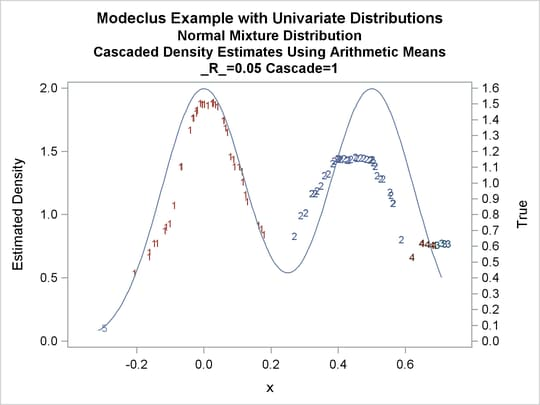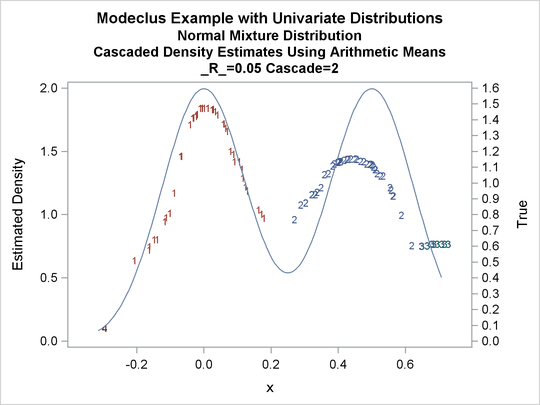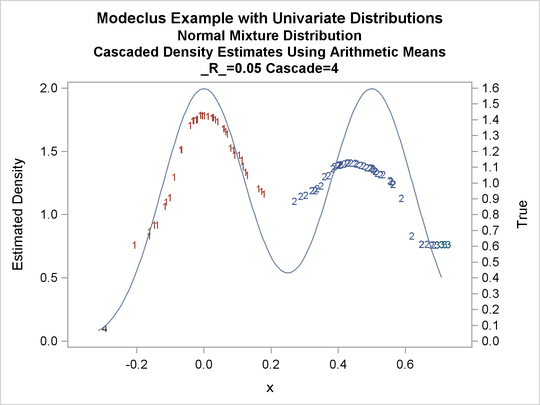# The MODECLUS Procedure

### Example 60.1 Cluster Analysis of Samples from Univariate Distributions

This example uses pseudo-random samples from a uniform distribution, an exponential distribution, and a bimodal mixture of two normal distributions. Results are presented in Output 60.1.1 through Output 60.1.18 as plots displaying both the true density and the estimated density, as well as cluster membership.

The following statements produce Output 60.1.1 through Output 60.1.4:

```title 'Modeclus Example with Univariate Distributions';
title2 'Uniform Distribution';

data uniform;
drop n;
true=1;
do n=1 to 100;
x=ranuni(123);
output;
end;
run;
```
```proc modeclus data=uniform m=1 k=10 20 40 60 out=out short;
var x;
run;

proc sgplot data=out noautolegend;
y2axis label='True' values=(0 to 2 by 1.);
yaxis values=(0 to 3 by 0.5);
scatter y=density x=x / markerchar=cluster group=cluster;
pbspline y=true x=x / y2axis nomarkers lineattrs=(thickness= 1);
by _K_;
run;
```
```proc modeclus data=uniform m=1 r=.05 .10 .20 .30 out=out short;
var x;
run;

proc sgplot data=out noautolegend;
y2axis label='True' values=(0 to 2 by 1.);
yaxis values=(0 to 2 by 0.5);
scatter y=density x=x / markerchar=cluster group=cluster;
pbspline y=true x=x / y2axis nomarkers lineattrs=(thickness= 1);
by _R_;
run;
```

Output 60.1.1: Cluster Analysis of Sample from a Uniform Distribution

 Modeclus Example with Univariate Distributions Uniform Distribution

The MODECLUS Procedure

Cluster Summary
K Number of
Clusters
Frequency of
Unclassified
Objects
10 6 0
20 3 0
40 2 0
60 1 0

Output 60.1.2: True Density, Estimated Density, and Cluster Membership by Various _K_ Values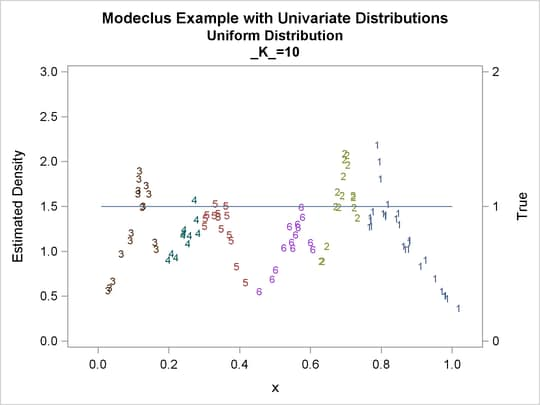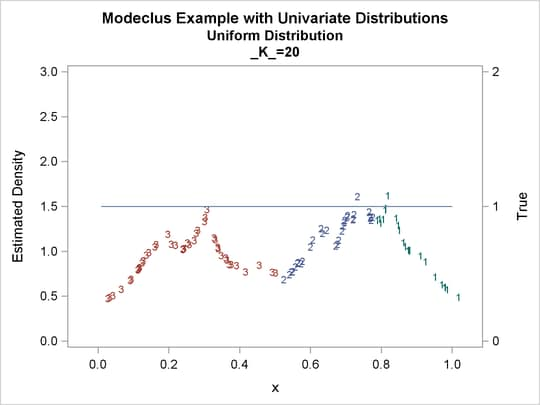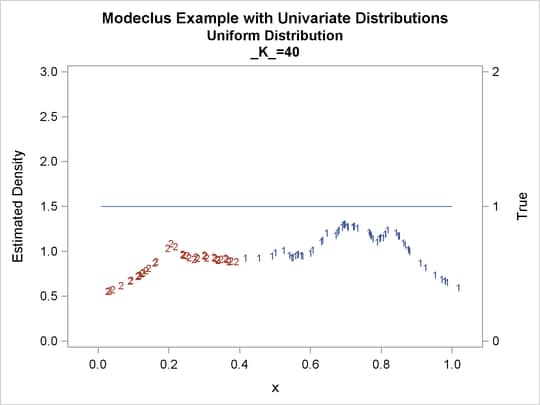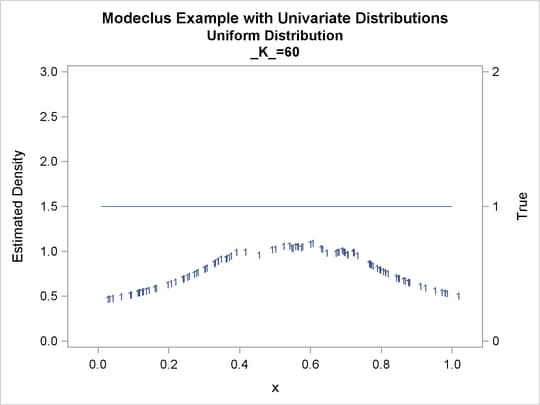Output 60.1.3: Cluster Analysis of Sample from a Uniform Distribution

 Modeclus Example with Univariate Distributions Uniform Distribution

The MODECLUS Procedure

Cluster Summary
R Number of
Clusters
Frequency of
Unclassified
Objects
0.05 4 0
0.1 2 0
0.2 2 0
0.3 1 0

Output 60.1.4: True Density, Estimated Density, and Cluster Membership by Various _R_ Values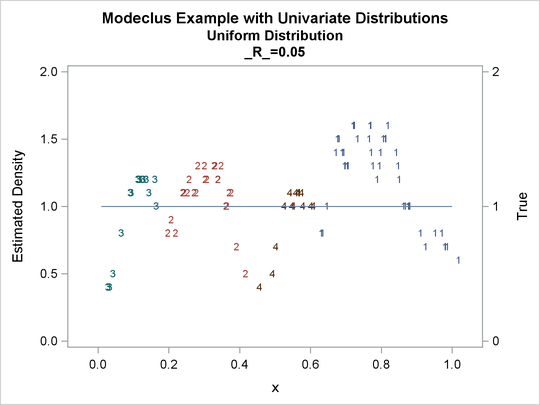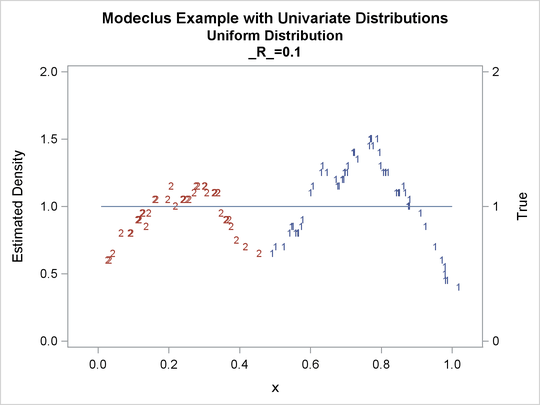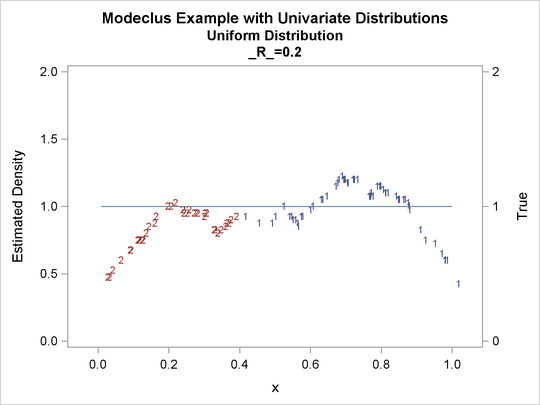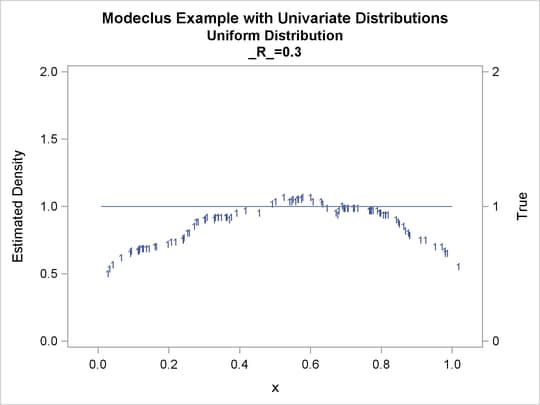The following statements produce Output 60.1.5 through Output 60.1.12:

```data expon;
title2 'Exponential Distribution';
drop n;
do n=1 to 100;
x=ranexp(123);
true=exp(-x);
output;
end;
run;
```
```proc modeclus data=expon m=1 k=10 20 40 out=out short;
var x;
run;

proc sgplot data=out noautolegend;
y2axis label='True' values=(0 to 1 by .5);
yaxis values=(0 to 2 by 0.5);
scatter y=density x=x / markerchar=cluster group=cluster;
pbspline y=true x=x / y2axis nomarkers lineattrs=(thickness= 1);
by _K_;
run;
```
```proc modeclus data=expon m=1 r=.20 .40 .80 out=out short;
var x;
run;

proc sgplot data=out noautolegend;
y2axis label='True' values=(0 to 1 by .5);
yaxis values=(0 to 1 by 0.5);
scatter y=density x=x / markerchar=cluster group=cluster;
pbspline y=true x=x / y2axis nomarkers lineattrs=(thickness= 1);
by _R_;
run;
```
```title3 'Different Density-Estimation and Clustering Windows';

proc modeclus data=expon m=1 r=.20 ck=10 20 40
out=out short;
var x;
run;

proc sgplot data=out noautolegend;
y2axis label='True' values=(0 to 1 by .5);
yaxis values=(0 to 1 by 0.5);
scatter y=density x=x / markerchar=cluster group=cluster;
pbspline y=true x=x / y2axis nomarkers lineattrs=(thickness= 1);
by _CK_;
run;
```
```title3 'Cascaded Density Estimates Using Arithmetic Means';

proc modeclus data=expon m=1 r=.20 cascade=1 2 4 am out=out short;
var x;
run;

proc sgplot data=out noautolegend;
y2axis label='True' values=(0 to 1 by .5);
yaxis values=(0 to 1 by 0.5);
scatter y=density x=x / markerchar=cluster group=cluster;
pbspline y=true x=x / y2axis nomarkers lineattrs=(thickness= 1);
run;
```

Output 60.1.5: Cluster Analysis of Sample from an Exponential Distribution

 Modeclus Example with Univariate Distributions Exponential Distribution

The MODECLUS Procedure

Cluster Summary
K Number of
Clusters
Frequency of
Unclassified
Objects
10 5 0
20 3 0
40 1 0

Output 60.1.6: True Density, Estimated Density, and Cluster Membership by Various _K_ Values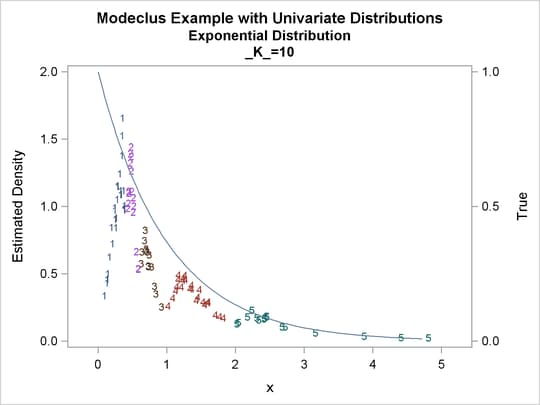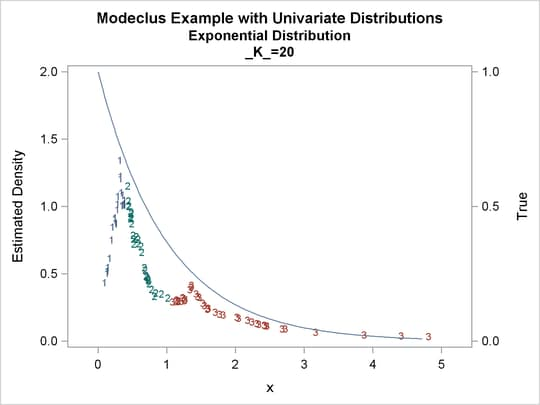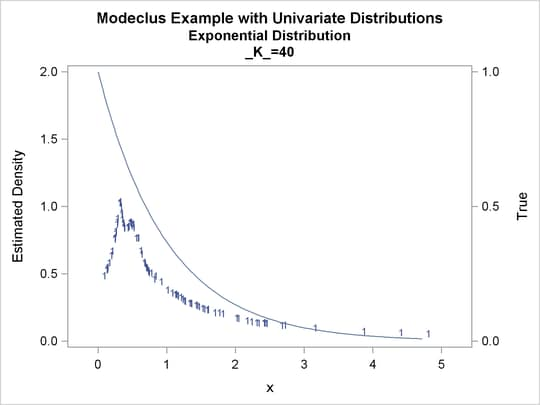Output 60.1.7: Cluster Analysis of Sample from an Exponential Distribution

 Modeclus Example with Univariate Distributions Exponential Distribution

The MODECLUS Procedure

Cluster Summary
R Number of
Clusters
Frequency of
Unclassified
Objects
0.2 8 0
0.4 6 0
0.8 1 0

Output 60.1.8: True Density, Estimated Density, and Cluster Membership by Various _R_ Values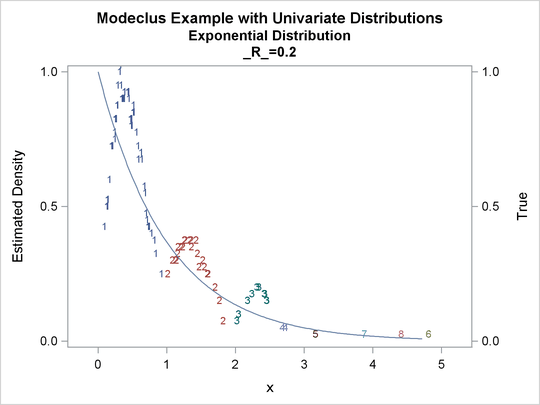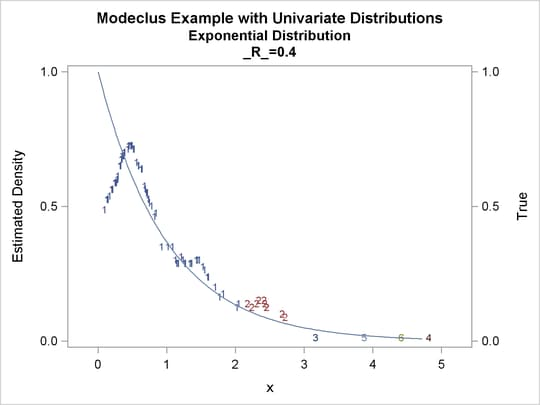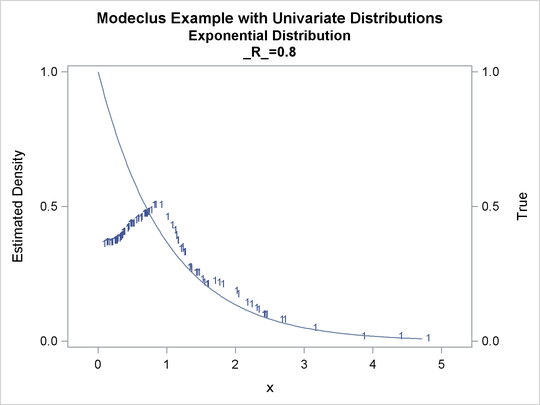Output 60.1.9: Cluster Analysis of Sample from an Exponential Distribution

 Modeclus Example with Univariate Distributions Exponential Distribution Different Density-Estimation and Clustering Windows

The MODECLUS Procedure

Cluster Summary
R CK Number of
Clusters
Frequency of
Unclassified
Objects
0.2 10 3 0
0.2 20 2 0
0.2 40 1 0

Output 60.1.10: True Density, Estimated Density, and Cluster Membership by _R_=0.2 with Various _CK_ Values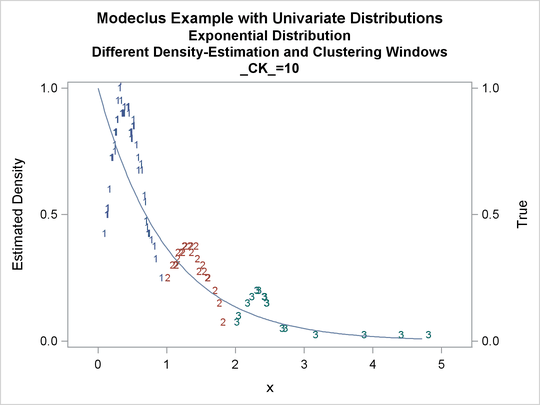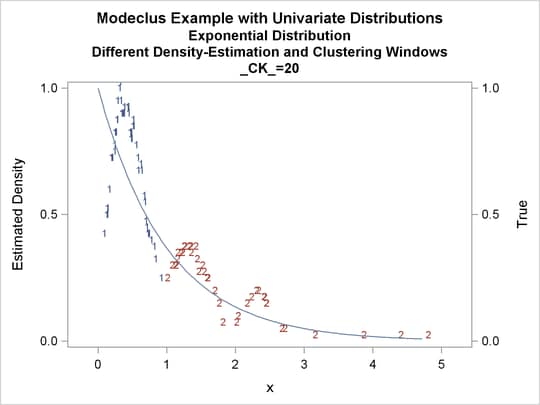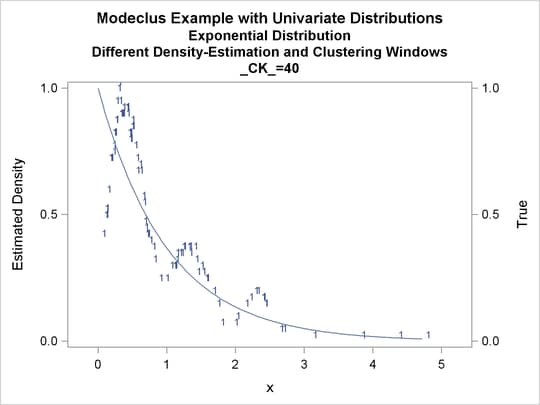Output 60.1.11: Cluster Analysis of Sample from an Exponential Distribution

 Modeclus Example with Univariate Distributions Exponential Distribution Cascaded Density Estimates Using Arithmetic Means

The MODECLUS Procedure

Cluster Summary
Clusters
Frequency of
Unclassified
Objects
0.2 1 8 0
0.2 2 8 0
0.2 4 7 0

Output 60.1.12: True Density, Estimated Density, and Cluster Membership by _R_=0.2 with Various _CASCAD_ Values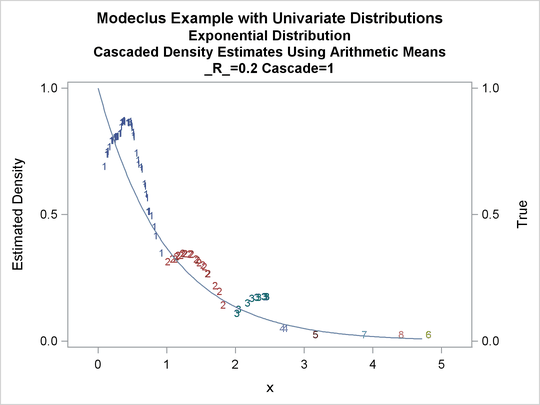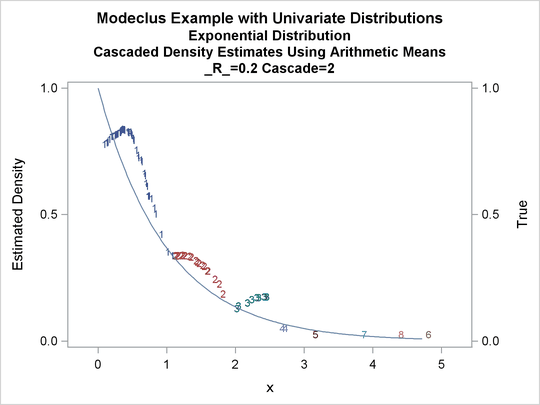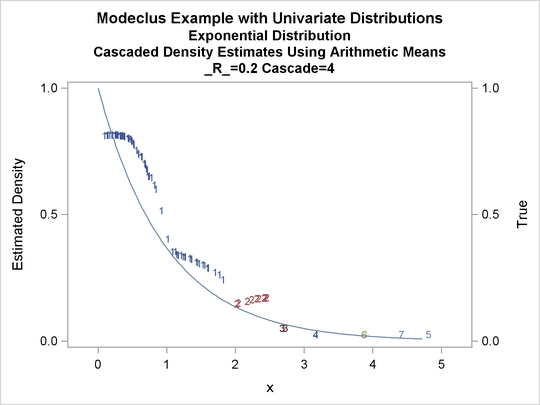The following statements produce Output 60.1.13 through Output 60.1.18:

```title2 'Normal Mixture Distribution';

data normix;
drop n sigma;
sigma=.125;
do n=1 to 100;
x=rannor(456)*sigma+mod(n,2)/2;
true=exp(-.5*(x/sigma)**2)+exp(-.5*((x-.5)/sigma)**2);
true=.5*true/(sigma*sqrt(2*3.1415926536));
output;
end;
run;
```
```proc modeclus data=normix m=1 k=10 20 40 60 out=out short;
var x;
run;

proc sgplot data=out noautolegend;
y2axis label='True' values=(0 to 1.6 by .1);
yaxis values=(0 to 3 by 0.5);
scatter y=density x=x / markerchar=cluster group=cluster;
pbspline y=true x=x / y2axis nomarkers lineattrs=(thickness= 1);
by _K_;
run;
```
```proc modeclus data=normix m=1 r=.05 .10 .20 .30 out=out short;
var x;
run;

proc sgplot data=out noautolegend;
y2axis label='True' values=(0 to 1.6 by .1);
yaxis values=(0 to 3 by 0.5);
scatter y=density x=x / markerchar=cluster group=cluster;
pbspline y=true x=x / y2axis nomarkers lineattrs=(thickness= 1);
by _R_;
run;
```
```title3 'Cascaded Density Estimates Using Arithmetic Means';

proc modeclus data=normix m=1 r=.05 cascade=1 2 4 am out=out short;
var x;
run;

proc sgplot data=out noautolegend;
y2axis label='True' values=(0 to 1.6 by .1);
yaxis values=(0 to 2 by 0.5);
scatter y=density x=x / markerchar=cluster group=cluster;
pbspline y=true x=x / y2axis nomarkers lineattrs=(thickness= 1);
run;
```

Output 60.1.13: Cluster Analysis of Sample from a Bimodal Mixture of Two Normal Distributions

 Modeclus Example with Univariate Distributions Normal Mixture Distribution

The MODECLUS Procedure

Cluster Summary
K Number of
Clusters
Frequency of
Unclassified
Objects
10 7 0
20 2 0
40 2 0
60 1 0

Output 60.1.14: True Density, Estimated Density, and Cluster Membership by Various _K_ Values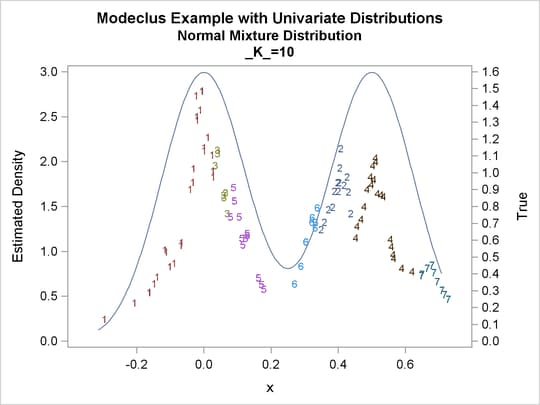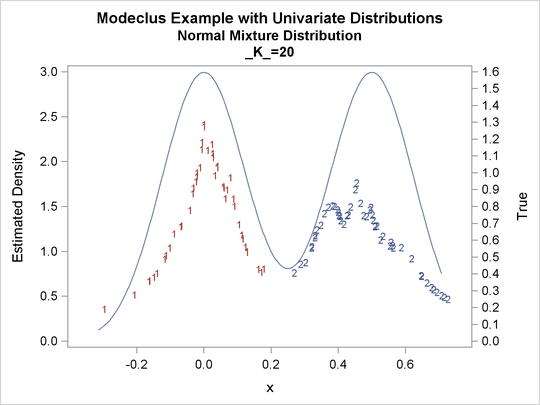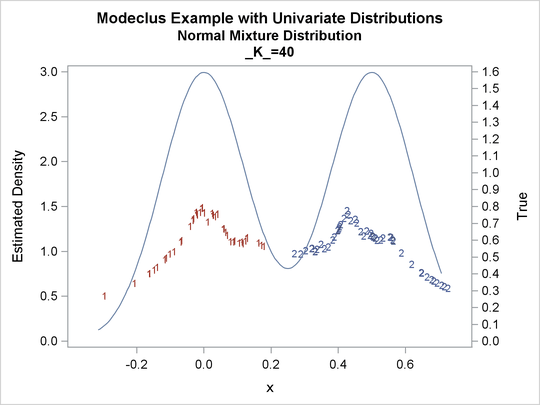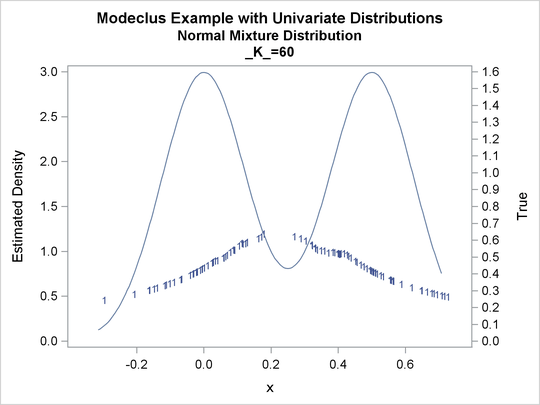Output 60.1.15: Cluster Analysis of Sample from a Bimodal Mixture of Two Normal Distributions

 Modeclus Example with Univariate Distributions Normal Mixture Distribution

The MODECLUS Procedure

Cluster Summary
R Number of
Clusters
Frequency of
Unclassified
Objects
0.05 5 0
0.1 2 0
0.2 2 0
0.3 1 0

Output 60.1.16: True Density, Estimated Density, and Cluster Membership by Various _R_= Values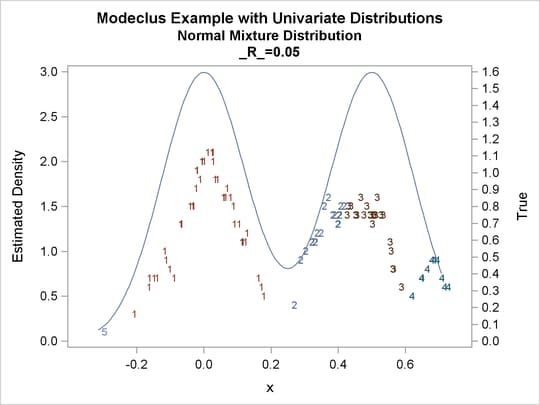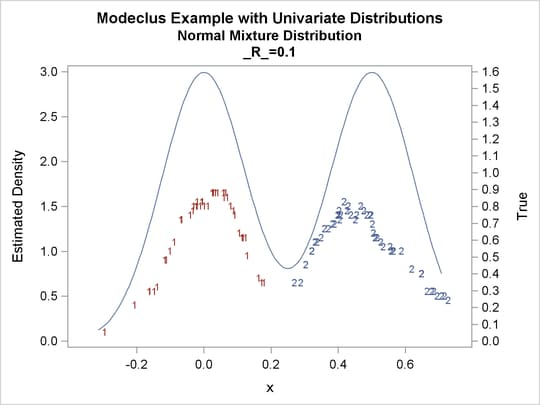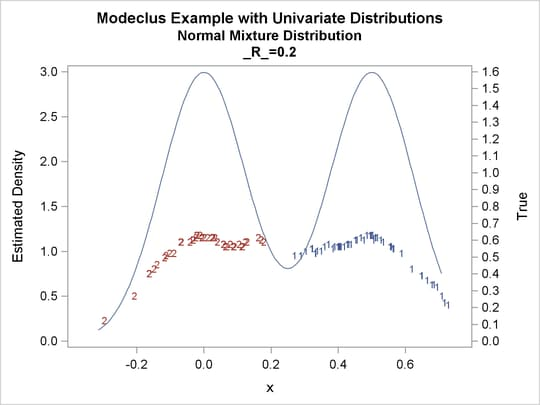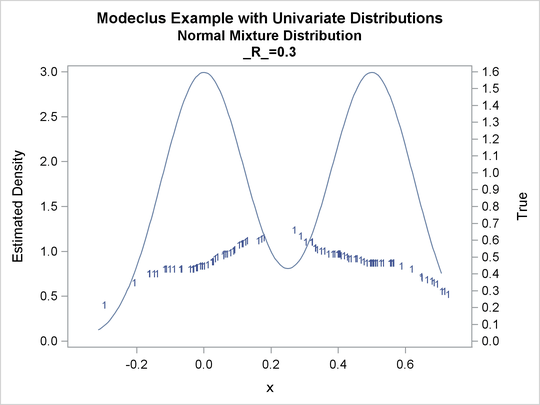Output 60.1.17: Cluster Analysis of Sample from a Bimodal Mixture of Two Normal Distributions

 Modeclus Example with Univariate Distributions Normal Mixture Distribution Cascaded Density Estimates Using Arithmetic Means

The MODECLUS Procedure

Cluster Summary
Clusters
Frequency of
Unclassified
Objects
0.05 1 5 0
0.05 2 4 0
0.05 4 4 0

Output 60.1.18: True Density, Estimated Density, and Cluster Membership by _R_=0.05 with Various _CASCAD_ Values